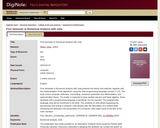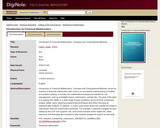Updating search results...

# 2 Results

View
Selected filters:
• DigiNoleConditional Remix & Share Permitted
CC BY-NC-SA
Rating
0.0 stars

First Semester in Numerical Analysis with Julia presents the theory and methods, together with the implementation of the algorithms using the Julia programming language (version 1.1.0). The book covers computer arithmetic, root-finding, numerical quadrature and differentiation, and approximation theory. The reader is expected to have studied calculus and linear algebra. Some familiarity with a programming language is beneficial, but not required. The programming language Julia will be introduced in the book. The simplicity of Julia allows bypassing the pseudocode and writing a computer code directly after the description of a method while minimizing the distraction the presentation of a computer code might cause to the flow of the main narrative.

Subject:
Mathematics
Material Type:
Textbook
Provider:
Florida State University
Provider Set:
DigiNole
Author:
Giray Ökten
04/23/2019Conditional Remix & Share Permitted
CC BY-NC-SA
Rating
0.0 stars

Introduction to Financial Mathematics: Concepts and Computational Methods serves as a primer in financial mathematics with a focus on conceptual understanding of models and problem solving. It includes the mathematical background needed for risk management, such as probability theory, optimization, and the like. The goal of the book is to expose the reader to a wide range of basic problems, some of which emphasize analytic ability, some requiring programming techniques and others focusing on statistical data analysis. In addition, it covers some areas which are outside the scope of mainstream financial mathematics textbooks. For example, it presents marginal account setting by the CCP and systemic risk, and a brief overview of the model risk. Inline exercises and examples are included to help students prepare for exams on this book.

Subject: